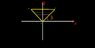# Moment of Inertia of a uniform triangle(involves integration)

• hms.tech
In summary, the student is trying to find the moment of inertia about one of the rod's sides. They use integration to find the exact moment of inertia and find that it is \frac{2m}{\sqrt{3}}\sum y^{3} Δy.f

## Homework Statement

The attachment shows an equilateral triangle of side length "2d"
It is a uniform triangle in the 2-D space.
Mass of triangle = M
I have to find the moment of inertia about one of its side . I am taking the side $\overline{AB}$ as the axis of rotation (hence i would be finding the Moment of inertia about this line)

The pink lines inside the triangle show an attempt to this problem.

## Homework Equations

>Integration
>area of triangle
>$I_{total}$ = $\sum I_{1} + I_{2} + I_{3}... I_{n}$
>β = 60 degrees

## The Attempt at a Solution

Let the mass per unit area of the triangle be = m
The triangle can be considered (approximated) to be composed of many small rods of width Δy and length "x" (where x varies as the equation of the line)

Now, the area of this rod is = 2xΔy
Hence mass of the rod = 2mxΔy
moment of inertia of one of the rod about $\overline{AB}$ = 2mx$y^{2}$Δy
The equation of the line $\overline{AC}$ is : y = tan(60)x = x$\sqrt{3}$

x = y/$\sqrt{3}$

Approximate moment of inertia = $\frac{2m}{\sqrt{3}}$$\sum y^{3} Δy$

we use integration to find the exact moment of inertia :

$I_{total}$ = $\frac{2m}{\sqrt{3}}$$\int (y)^{3} dy$

the limits of integration are from 0$\rightarrow\sqrt{3}d$

Area of triangle = $\sqrt{3}$$d^{2}$
Moment of inertia of the triangle = $\frac{3Md^2}{2}$

The problem is that this answer is wrong, and the correct answer is : $Md^2/2$ [which i have deduced by flipping the triangle (i will post an attachment of the way the triangle was oriented which lead me to the correct answer] However the method for both was same, and the orientation of the triangle should never affect the Moment of inertia as long as it is taken about the same axis .

#### Attachments

•Untitled.png
3.8 KB · Views: 737
Last edited:
I suspect that the answer lies in precisely what you are NOT showing: exactly how you calculated the moment of the "flipped" triangle. If you flip the triangle so that the vertex is at (0, 0), the side you are rotating around will be $y= \sqrt{3}d$.

I don't know what u mean by that, i don't understand in what way are u rotating,
but i rotated it in such a way to give this figure. using this diagram (attached to this post) i got the right answer

Even though i used the same method as before (of course the x will be replaced with y)

It is surprising that one of the answers is wrong by the exact same method .

#### Attachments

Last edited:
Can someone explain why does the first method give me a wrong answer .

Bump .. .. ..

Bump . . .

I guess, you've calculated the moment of inertia around the middle axis of the triangle instead of around one of its sides.

The area of the triangle is $A=\frac{\sqrt{3}}{4} a^2=\sqrt{3} d^2$ with $a=2d$ the length of the triangle's sides.

To make the calculation easier, I put one side around the $x$ axis of a cartesian coordinate system, which we also take as the axis of rotation, and one of its corners on the $y$ axis.

Then possible $y$ values are in the interval $(0,\sqrt{3} d)$ and for each $y$-value the $x$ values are in the interval $(y/\sqrt{3}-d,d-y/\sqrt{3})$.

The moment of inertia is thus given by the area integral
$$I=\frac{m}{A} \int_{A} \mathrm{d} F \; y^2=\frac{m}{\sqrt{3} d^2} \int_0^{\sqrt{3} d} \mathrm{d} y 2 \int_0^{d-y/\sqrt{3}} \mathrm{d} x \; y^2.$$
The integration is straight forward. With the $x$ integral done you get
$$I=\frac{2m}{\sqrt{3} d^2} \int_0^{\sqrt{3}d} \mathrm{d} y \; \left(d y^2-\frac{y^3}{\sqrt{3}} \right )$$
After the integration and some algebra you finally get
$$I=\frac{m d^2}{2}.$$

Since vanhees has done the algebra whilst I was asleep, (thanks mate) there is little left for me to do except add value.

Have your heard of the transfer theorem/ parallel axis theorem/theorem of Pappus?

You need to always check what axis of rotation is being used. Standard tables often refer to

Ixx and Iyy

These are taken through the centroid of the figure., not about an edge or other axis.

General integration of an elemental area will always be about a stated axis or it is valueless.

Edit : I mentioned product of inertia before, have you met this term?
The following thread may be of value

go well

Last edited:
Hi hms, I'm a new member and this is my first post,

You did everything right except for one thing. Here is the reason you didn't get an answer of $\frac{1}{2}$$M$$d^{2}$ in your initial post. The $y^{2}$ represents the square of the distance between your rods and AB which is the error (further study of your diagram shows this). Replace your $y^{2}$ with $\left(\sqrt{3}d-y\right)^{2}$ and then continue as before and you will get the right answer.

I would like to write out the whole process for you but it would take ages in latex.

Please let me know if this works for you, as it worked for me when I solved it on paper using this method and got the right answer of $\frac{1}{2}$$M$$d^{2}$.

Regards,
IZ

Last edited: